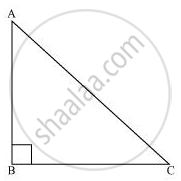# Show that in a right angled triangle, the hypotenuse is the longest side. - Mathematics

Show that in a right angled triangle, the hypotenuse is the longest side.

#### SolutionLet us consider a right-angled triangle ABC, right-angled at B.

In ΔABC,

∠A + ∠B + ∠C = 180° (Angle sum property of a triangle)

∠A + 90º + ∠C = 180°

∠A + ∠C = 90°

Hence, the other two angles have to be acute (i.e., less than 90º).

∴ ∠B is the largest angle in ΔABC.

⇒ ∠B > ∠A and ∠B > ∠C

⇒ AC > BC and AC > AB

[In any triangle, the side opposite to the larger (greater) angle is longer.]

Therefore, AC is the largest side in ΔABC.

However, AC is the hypotenuse of ΔABC. Therefore, hypotenuse is the longest side in a right-angled triangle.

Concept: Inequalities in a Triangle
Is there an error in this question or solution?
Chapter 7: Triangles - Exercise 7.4 [Page 132]

#### APPEARS IN

NCERT Class 9 Maths
Chapter 7 Triangles
Exercise 7.4 | Q 1 | Page 132

Share